Courses

# Test: Counting In Groups - 2

## 20 Questions MCQ Test Mathematics (Maths) for Class 2 | Test: Counting In Groups - 2

Description
This mock test of Test: Counting In Groups - 2 for Class 2 helps you for every Class 2 entrance exam. This contains 20 Multiple Choice Questions for Class 2 Test: Counting In Groups - 2 (mcq) to study with solutions a complete question bank. The solved questions answers in this Test: Counting In Groups - 2 quiz give you a good mix of easy questions and tough questions. Class 2 students definitely take this Test: Counting In Groups - 2 exercise for a better result in the exam. You can find other Test: Counting In Groups - 2 extra questions, long questions & short questions for Class 2 on EduRev as well by searching above.
QUESTION: 1

Solution:
QUESTION: 2

Solution:
QUESTION: 3

### The cost of 5 oranges and 6 apples are Rs. 45 and Rs. 67 respectively. What is the total cost of both quantity of fruits?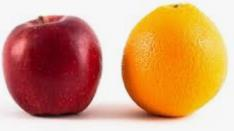Solution:
QUESTION: 4

Add the following: 34 + 15 + 13 = ?

Solution:
QUESTION: 5

The length and width of a plane figure is 37 22 metres respectively Find the length of the boundary of the figure if there is another pair of same length and width.

Solution:
QUESTION: 6

Add: 5 + 6 + 8 + 0

Solution:
QUESTION: 7

Add the total money which is paid for the cost of the given articles.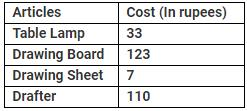Solution:
QUESTION: 8

There are 56 women and 50 men at an event. How many people are there in all if no child are there at the event?Solution:
QUESTION: 9

Add first four numbers from the table of 2.

Solution:
QUESTION: 10

Add: 45 + 32 + 56 + 40.

Solution:
QUESTION: 11

There are 322 people at a place and 329 people join them. How many people are there at the place?Solution:
QUESTION: 12

Solution:
QUESTION: 13

There are 56 female, 80 male and 34 children in a park. How many people are there in the park?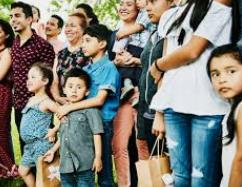Solution:
QUESTION: 14

Count and add total number of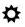in both the figures given below.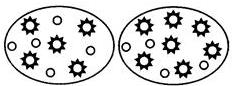Solution:
QUESTION: 15

Solution:
QUESTION: 16

Solution:
QUESTION: 17

Consider the following statements:
Statement 1: The addition of a number with zero is the number itself,
Statement 2: The addition of a number with zero is the zero.

Q. Which one of the following is correct about the above statements?

Solution:
QUESTION: 18

Add: 5 hours + 30 minutes + 50 hours + 12 minutes.

Solution:
QUESTION: 19

Peter gets Rs.367 from his father, Rs. 56 from his uncle and Rs.100 from his mother. How much money he has now?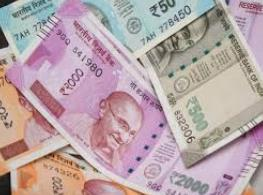Solution:
QUESTION: 20

Fill in the appropriate number in the given below.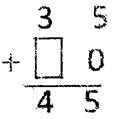Solution: